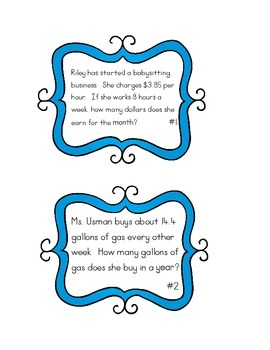# Multiplying Decimals Task Cards Word ProblemsSubject
Resource Type
File Type
PDF (288 KB|15 pages)
Standards
\$2.00
• Product Description
• Standards
This set of 24 multiplying decimals task cards for your students to practice their decimal computational skills. Use them as math centers, SCOOT!, or any way that works for your instructional style. There are 24 total real world tasks cards, 15 where students multiply a whole number by a decimal and 9 multiplying a decimal by a decimal. - ALL set with real world story problems. Answer sheet is included. For assessment, you may want to check out our FSA assessment for NBT7!
Add, subtract, multiply, and divide decimals to hundredths, using concrete models or drawings and strategies based on place value, properties of operations, and/or the relationship between addition and subtraction; relate the strategy to a written method and explain the reasoning used.
Total Pages
15 pages